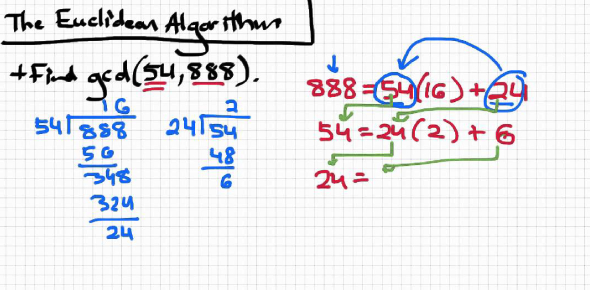How would you prove the Pythagoras theorem through Euclid's method? - ProProfs DiscussTopicsMoreProductsMore+ Ask Question

# How would you prove the Pythagoras theorem through Euclid's method?

Asked by K. Tanaka, Last updated: Mar 18, 2020

###Request 0FollowShareAnswer AnonymouslyAnswer LaterCopy Link#### John F. connor

Traveler, Avid Reader, Free as a bird

John F. connor, Content Marketing executive, MA, Minsk,PolandEuclid had a method known as Euclidean algorithm. The main point of using this algorithm is to determine the greatest common divisor. Two numbers are given and using this method, you can figure out the highest number that can evenly be divided into those two numbers. Like many math problems, two methods or theorems can be applied.In proofs, they may both be used. One way to use both would be in a problem where you are trying to use other ways to find out one of the sides of a right triangle. You may end up using the Euclid’s method. Then you could use the theorem to calculate the hypotenuse of the long side of the triangle. Then solve the problem.Search for Google imagesSelect a recommended image
Upload from your computerCancelSearch for Google imagesSelect a recommended image
Upload from your computerCancelSearch for Google imagesSelect a recommended image
Upload from your computerCancel